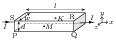# Hall Effect

## Problems from IIT JEE

A paragraph based problem was asked in IIT JEE 2015. The problem is given below,

Problem (IIT JEE 2015):

Paragraph:In a thin rectangular metallic strip a constant current $I$ flows along the positive $x$-direction, as shown in the figure. The length, width and thickness of the strip are $l$, $w$ and $d$, respectively. A uniform magnetic field $\vec{B}$ is applied on the strip along the positive $y$-direction. Due to this, the charge carriers experience a net deflection along the $z$-direction. This results in accumulation of charge carriers on the surface PQRS and appearance of equal and opposite charges on the face opposite to PQRS. A potential difference along the $z$-direction is thus developed. Charge accumulation continues until the magnetic force is balanced by the electric force. The current is assumed to be uniformly distributed on the cross section of the strip and carried by electrons.

Question 1: Consider two different metallic strips (1 and 2) of the same material. Their lengths are the same, widths are $w_1$ and $w_2$ and thicknesses are $d_1$ and $d_2$, respectively. Two points K and M are symmetrically located on the opposite faces parallel to the $x\text{-}y$ plane (see figure). $V_1$ and $V_2$ are the potential differences between K and M in strips 1 and 2, respectively. Then, for a given current $I$ flowing through them in a given magnetic field strength $B$, the correct statement(s) is(are),

1. If $w_1=w_2$ and $d_1=2d_2$, then $V_2=2V_1$.
2. If $w_1=w_2$ and $d_1=2d_2$, then $V_2=V_1$.
3. If $w_1=2w_2$ and $d_1=d_2$, then $V_2=2V_1$.
4. If $w_1=2w_2$ and $d_1=d_2$, then $V_2=V_1$.

Solution:The charge carriers in a metallic strip are electrons. The electrons moves in a direction opposite to the current flow with a drift velocity given by, \begin{align} v_d=\frac{I}{neA}=\frac{I}{newd}, \end{align} where $n$ is the number density of the electrons (number of electrons per unit volume), $e$ is the electronic charge, and $A=wd$ is the cross-sectional area of the strip. The electrons move with velocity $\vec{v}_d=-v_d\,\hat\imath$ in a uniform magnetic field $\vec{B}=B\hat\jmath$. Thus, magnetic force on the moving electrons is, \begin{align} \vec{F}_m=q\vec{v}_d\times\vec{B}=(-e) (-v_d\,\hat\imath)\times(B\,\hat\jmath)=ev_d B\,\hat{k}=\frac{IB}{nwd}\,\hat{k}. \end{align} Note that an electron has a negative charge $q=-e$. From above equation, the electrons start moving towards the face PQRS leading to the negative potential at this face. This potential establishes an electric field in a direction from K to M. If $V$ is the potential difference between these faces then electric field is $\vec{E}=(V/w)\,\hat{k}$. The electric force on the electrons is, \begin{align} \vec{F}_e=q\vec{E}=(-e)\frac{V}{w}\,\hat{k}=-\frac{eV}{w}\,\hat{k}. \end{align} The accumulation of electrons on face PQRS continues till the net force on the electrons become zero (equilibrium condition). Use equations to get the potential difference in equilibrium condition, \begin{align} V=\frac{IB}{ned}. \end{align} From this equation, $V$ depends on $d$ and not on $w$. Thus, if $d_1=d_2$ then $V_2=V_1$ and if $d_1=2d_2$ then $V_2=2V_1$. Thus, A and D are correct answers.

Question 2: Consider two different metallic strips (1 and 2) of same dimensions (length $l$, width $w$ and thickness $d$) with carrier densities $n_1$ and $n_2$, respectively. Strip 1 is placed in magnetic field $B_1$ and strip~2 is placed in magnetic field $B_2$, both along positive $y$-directions. Then $V_1$ and $V_2$ are the potential differences developed between $K$ and $M$ in strips 1 and 2, respectively. Assuming that the current $I$ is the same for both the strips, the correct options(s) is(are),

1. If $B_1=B_2$ and $n_1=2n_2$, then $V_2=2V_1$.
2. If $B_1=B_2$ and $n_1=2n_2$, then $V_2=V_1$.
3. If $B_1=2B_2$ and $n_1=n_2$, then $V_2=0.5V_1$.
4. If $B_1=2B_2$ and $n_1=n_2$, then $V_2=V_1$.

Solution: The ratio of potential difference in two strips is given by, \begin{align} \frac{V_2}{V_1}=\frac{B_2}{B_1}\frac{n_1}{n_2}. \end{align} Substitute $B_1=B_2$ and $n_1=2n_2$ in equation to get $V_2=2V_1$. Substitute $B_1=2B_2$ and $n_1=n_2$ in above equation to get $V_2=0.5V_1$. Thus, A and C are correct answers.